# How many grams of dry NH4Cl need to be added to 2.50 L of a 0.500 M solution of ammonia, NH3, to prepare a buffer soluti...

How many grams of dry NH4Cl need to be added to 2.50 L of a 0.500 M solution of ammonia, NH3, to prepare a buffer solution that has a pH of 8.74? Kb for ammonia is 1.8*10^-5.

Concepts and reason

Buffer:

A buffer is a chemical solution that contains a weak acid/base mixed with its conjugate base/acid (as in the form of salts). It can resist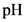change when a small amount of a strong acid/base is added to it. The acidic buffer solution is made up of less than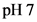and the alkali buffer solution is made up of more than.

Fundamentals

Henderson-Hasselbalch equation is a mathematical expression which can be used to calculate theof buffer solutions.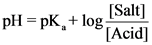Concentration of acid and base can be calculated as follows:Write the Henderson-Hasselbalch equation of basic buffer calculation.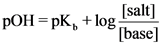Concentration of acid and base can be calculated as follows:The reaction for given buffer solution can be written as follows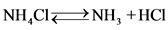Given data: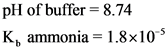So,Then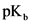of given solution can be calculated as followsHenderson-Hasselbalch was used for calculating the mass of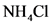as given below, the values of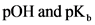were substituted in Henderson-Hasselbalch equation.ThenFinally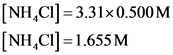The mole of ammonium chloride was calculated as follows: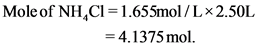The mass of ammonium chloride is calculated as follows: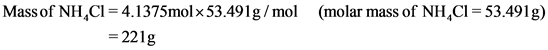Ans:

The mass of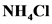is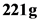.

##### Add Answer of: How many grams of dry NH4Cl need to be added to 2.50 L of a 0.500 M solution of ammonia, NH3, to prepare a buffer soluti...
More Homework Help Questions Additional questions in this topic.